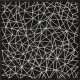# Dihedral Groups and Automorphisms, Part 1

Welcome to the second two-post series on AZC! The evil secret plot of this series is to make group cohomology seem interesting for those who have not seen much group cohomology. To do this, we will dissect the dihedral group, which most math majors have probably seen as undergrads. However, to be thorough, the first post (i.e. this one) will described the dihedral group, and only in the second (will be posted soon) will we bring in group cohomology.

The finite dihedral groups are a good, concrete example of finite groups because they are not abelian and yet are not too convoluted for a blog post. There are many ways to define the dihedral groups, but the one that perhaps gives the most context and motivation is the definition in terms of symmetry of equilateral polygons in the Euclidean plane.

For an integer $n \geq 3$, the dihedral group $D_n$ is the symmetry group of rotations and reflections of the Euclidean planar regular $n$-gon. Let us look at the case $n = 3$ in a bit more detail. By labelling the vertices of an equilateral triangle with $\{a,b,c\}$, we can deduce that $D_3$ has order six. In fact, any fixed letter can be rotated to any given position, and then the remaining two letters can be permuted via a reflection, so all of the $3! = 6$ possible configurations obtainable with reflections and rotations.

Actually, by this reasoning $D_3$ is in fact $S_3$, the permutation group on $3$ symbols, but in general $D_n$ is not $S_n$. Just think of a hexagon for instance, where one vertex will always be adjacent to the same two vertices, which restricts the possible permutations.

### Presentations

The dihedral group is in fact generated by a rotation by an angle of $2\pi/n$ and any reflection that actually preserves symmetry. A bit more geometric reasoning, which is strongly recommended as a good exercise, shows that the dihedral group $D_n$ is in fact given by the presentation

$\langle r, s | r^n, s^2, sr = r^{-1}s\rangle.$

### Semidirect Products

There is another interesting way to write the dihedral group $D_n$ as a semidirect product of two cyclic groups, one acting on the other. We will use the notation $C_m$ to denote the cyclic group of order $m$.

Let us define first the semidirect product. For a group $G$ and a $G$-module $A$ (that is, an abelian group $A$ with a $G$-action), the semidirect product $A\rtimes G$ is the group with underlying set the cartesian product $A\times G$, and with multiplication defined by $(a,g)(b,h) = (a + gb, gh)$. This is indeed a group (exercise) with identity $(0,1)$ and with the inverse of $(a,g)$ is $(-g^{-1}a,g^{-1})$. Both $A$ and $G$ are canonical subgroups, and there is a short exact sequence

$0\to A\to A\rtimes G\to G\to 1.$

Note that there is a more general notion of semidirect product of two subgroups (one normal) of a group, but we shall not need this here. The interested reader should consult any introductory group theory text.

### Back to the Dihedral Group

It turns out now that we didn't just introduce semidirect products because they are cool (even though they are), but because the dihedral group can be realized nicely as the semidirect product $C_n\rtimes C_2$ with $C_m$ as a $C_2$ module. To simplify notation, let us write $C_2 = \{ 1, \sigma\}$. Now, we give $C_m$ a $C_2$-module structure by the action $\sigma*k = -k$. Now we claim that $D_{n} \cong C_n\rtimes C_2$.

Proof. We use that $D_{n}$ is isomorphic to the presented group

$G = \langle r, s | r^n, s^2, sr = r^{-1}s\rangle$

and define $\varphi:G\to C_n\rtimes C_2$ by $r\mapsto (1,1)$ and $s\mapsto (0,\sigma)$. This is indeed a group homomorphism since it is compatible with the relations in the presentation for $G$. This homomorphism is surjective because $\varphi(r^k) = (k,1)$ and $\varphi(s) = (0,\sigma)$, and these generate $C_n\rtimes C_2$. Since $G$ and $C_n\rtimes C_2$ have the same cardinality, it is also injective.$\blacksquare$

### What's Next?

So we have seen the dihedral group in three different forms: a geometric description (a group acting on Euclidean space), a group given by a presentation, and a semidirect product, the last description being the most algebraic. In Part 2 of this series, we will introduce a bit of group cohomology and use it to prove an interesting property of the dihedral groups $D_n$ for which $n$ is even. Stay tuned!

### 1 Comment

•Maxime says:

I find the semidirect product interpretation quite geometric in nature. It is essentially capturing the idea that the relflexion s is acting by conjugation on the rotation generator srs^-1=r^-1 in the first presentation you give. Looking forward to the next post!